Skip to content

# Layout

## Table of Contents

The Elements Library provides a comprehensive set of elements for laying out elements in the view.

## Limits

Each element provides information on how it wants to be sized by means of `min` and `max` limits: a hint that determine its actual extent and how it will be placed in the view depending on available window space. The element’s `limits` member function determines the minimum and maximum extents of an element:

``````view_limits limits(basic_context const& ctx) const;
``````

See Context for information about `basic_context`. `view_limits` is a struct that gives us the `min` and `max` information:

``````struct view_limits
{
point    min = { 0.0, 0.0 };
point    max = { full_extent, full_extent };
};
``````

For the purpose of this document, we will use these terms and expressions:

limits
The limits of an element
limits.min
The minimum limits of an element
limits.min.x
The minimum horizontal limit of an element
limits.min.y
The minimum vertical limit of an element
limits.max
The maximum limits of an element
limits.max.x
The maximum horizontal limit of an element
limits.max.y
The maximum vertical limit of an element
horizontal limits
(limits.min.x, limits.max.x)
vertical limits
(limits.min.y, limits.max.y)
minimum limits
(limits.min.x, limits.min.y)
maximum limits
(limits.max.x, limits.max.y)
minimum horizontal limit
limits.min.x
maximum horizontal limit
limits.max.x
minimum vertical limit
limits.min.y
maximum vertical limit
limits.max.y

By default, an element has full limits: it is infinitely resizable:

``````constexpr view_limits full_limits = {
{ 0.0, 0.0 }
, { full_extent, full_extent }
};
``````

An element with `full_limits` can be resized from an empty point (zero x and y size) up to the full extent of the screen, and beyond (if possible).`full_extent` is actually an implementation defined huge number near the maximum limits of the coordinate’s data type (also implementation defined).

• An element has a fixed horizontal size if this expression is true: `limits.min.x == limits.max.x`.

• An element has a fixed vertical size if this expression is true: `limits.min.y == limits.max.y`.

#### Examples

``````{ { 100, 100 }, { 100, 100 } }; // Fixed size
``````
``````{ { 100, 100 }, { 100, full_extent } }; // Fixed width, flexible height
``````
``````{ { 100, 100 }, { 100, 200 } }; // Fixed width, semi-flexible height (100 to 200)
``````

## Size Elements

Size elements override the limits of an enclosed element. There is a comprehensive list of size related elements in the Elements Library that can be used for various purposes. This section catalogues all the available size elements.

### limit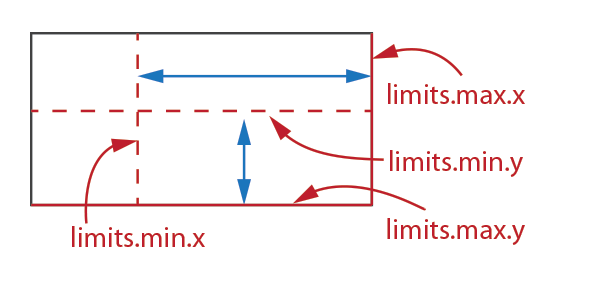Overrides the limits of an element.

#### Expression

``````limit(limits, subject)
``````

#### Notation

 `limits` Instance of `view_limits` `subject` Instance of `Element`

#### Semantics

1. The limits of `subject` will be set to the specified `limits` constrained by the natural limits of the `subject` (the natural limits of the element will not be violated).
2. Returns instance of `Proxy`.

### fixed_sizeFixes the size of an enclosed element (`subject`).

#### Expression

``````fixed_size({ width, height }, subject)
``````

#### Notation

 `width`, `height` `float` `subject` Instance of `Element`

#### Semantics

1. `subject` will be laid out with a fixed `width` and `height`, constrained by the natural limits of the `subject` (the natural limits of the element will not be violated).
2. Returns instance of `Proxy`.

### hsizeFixes the horizontal size of an enclosed element (`subject`).

#### Expression

``````hsize(width, subject)
``````

#### Notation

 `width` `float` `subject` Instance of `Element`

#### Semantics

1. `subject` will be laid out with a fixed `width`, constrained by the natural horizontal limits of the `subject` (the natural horizontal limits of the element will not be violated).
2. The natural vertical limits of `subject` will not be affected.
3. Returns instance of `Proxy`.

### vsize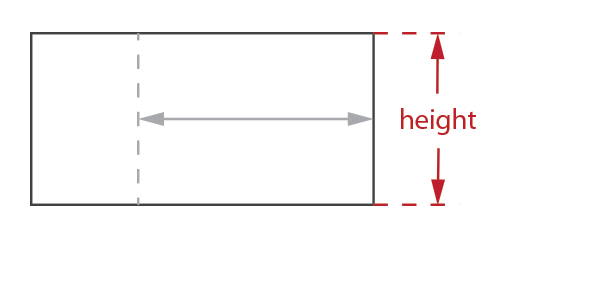Fixes the vertical limits of an enclosed element (`subject`).

#### Expression

``````vsize(height, subject)
``````

#### Notation

 `height` `float` `subject` Instance of `Element`

#### Semantics

1. `subject` will be laid out with a fixed `height`, constrained by the natural vertical limits of the `subject` (the natural vertical limits of the element will not be violated).
2. The natural horizontal limits of `subject` will not be affected.
3. Returns instance of `Proxy`.

### min_sizeOverrides the minimum limits of an enclosed element (`subject`).

#### Expression

``````min_size({ width, height }, subject)
``````

#### Notation

 `width`, `height` `float` `subject` Instance of `Element`

#### Semantics

1. The minimum limits of `subject` will be set to the specified `width` and `height`, constrained by the natural minimum limits of the `subject`.
2. the natural minimum limits of the element will not be violated.
3. Returns instance of `Proxy`.

### hmin_size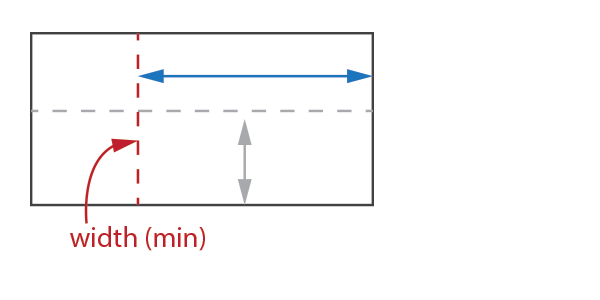Overrides the minimum horizontal limit of an enclosed element (`subject`).

#### Expression

``````hmin_size(width, subject)
``````

#### Notation

 `width` `float` `subject` Instance of `Element`

#### Semantics

1. The minimum horizontal limit of `subject` will be set to the specified `width` constrained by the natural horizontal minimum limits of the `subject`.
2. The natural horizontal minimum limits of the element will not be violated.
3. Returns instance of `Proxy`.

### vmin_size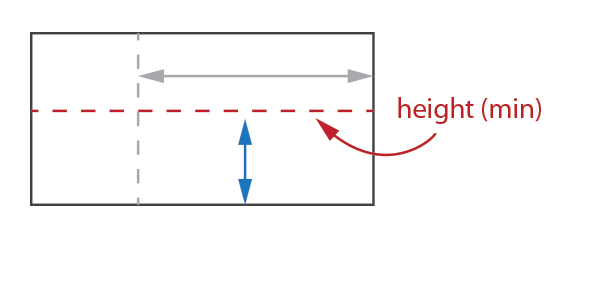Overrides the minimum vertical limit of an enclosed element (`subject`).

#### Expression

``````vmin_size(height, subject)
``````

#### Notation

 `height` `float` `subject` Instance of `Element`

#### Semantics

1. The minimum vertical limit of `subject` will be set to the specified `height` constrained by the natural vertical minimum limits of the `subject`.
2. The natural vertical minimum limits of the element will not be violated.
3. Returns instance of `Proxy`.

### max_size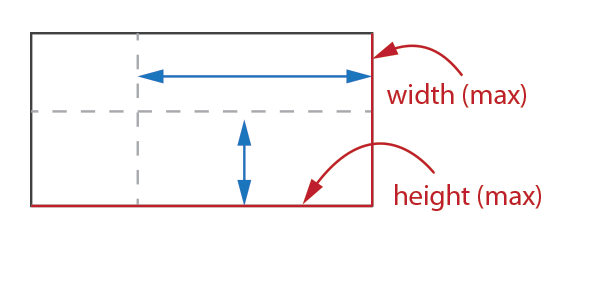Overrides the maximum limits of an enclosed element (`subject`).

#### Expression

``````max_size({ width, height }, subject)
``````

#### Notation

 `width`, `height` `float` subject Instance of `Element`

#### Semantics

1. The maximum limits of `subject` will be set to the specified `width` and `height`, constrained by the natural maximum limits of the `subject`.
2. The natural maximum limits of the element will not be violated.
3. Returns instance of `Proxy`.

### hmax_size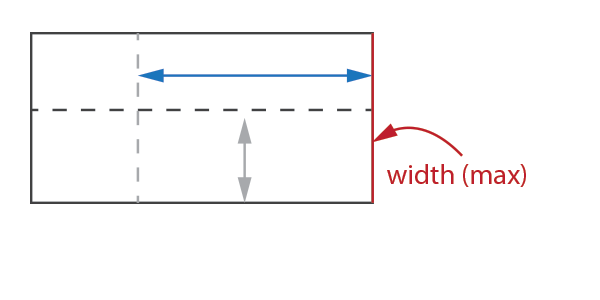Overrides the maximum horizontal limit of an enclosed element (`subject`).

#### Expression

``````hmax_size(width, subject)
``````

#### Notation

 `width` `float` `subject` Instance of `Element`

#### Semantics

1. The maximum horizontal limit of `subject` will be set to the specified `width` constrained by the natural maximum horizontal limit of the `subject`.
2. The natural maximum horizontal limit of the element will not be violated.
3. Returns instance of `Proxy`.

### vmax_size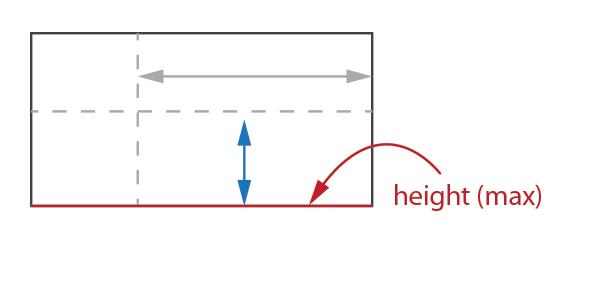Overrides the maximum vertical limit of an enclosed element (`subject`).

#### Expression

``````vmax_size(height, subject)
``````

#### Notation

 `height` `float` `subject` Instance of `Element`

#### Semantics

1. The maximum vertical limit of `subject` will be set to the specified `height` constrained by the natural maximum vertical limit of the `subject`
2. The natural maximum vertical limit of the element will not be violated.
3. Returns instance of `Proxy`.

## Stretch Elements

Resizable elements are elements with minimum limits that are less than the maximum limits in either x or y dimensions or both. Resizable elements can therefore stretch if there is extra available space allocated to it beyond its minimum limit. When two or more resizable elements are placed side by side in an htile or vtile, the element’s “stretchiness” determines how much extra space is given to it. The element’s “stretchiness” is determined by the element’s `stretch` member function:

``````virtual view_stretch stretch() const;
``````

where `view_stretch` is a struct declared as:

``````struct view_stretch
{
float    x = 1.0;
float    y = 1.0;
};
``````

The `view_stretch` `x` and `y` members determine how much an element can stretch in the x or y dimensions. The default is 1.0. A stretchiness value of 2.0 means that the element is able to stretch twice as much compared to its siblings in an htile or vtile, assuming they have the default 1.0 stretchiness.

### hstretch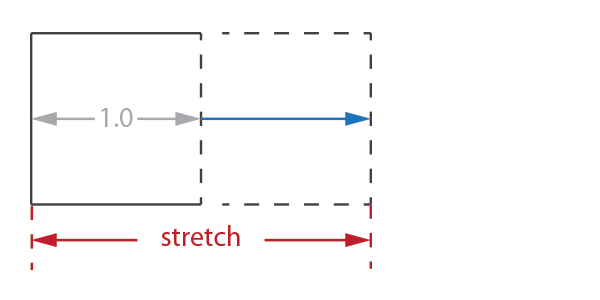Overrides the horizontal stretchiness of an an enclosed element (`subject`).

#### Expression

``````hstretch(stretch, subject)
``````

#### Notation

 `stretch` A scalar value `subject` Instance of `Element`

#### Semantics

1. The `subject` will assume the given `stretch` value.
2. The stretch value has no effect to elements with fixed horizontal size.
3. Returns instance of `Proxy`.

For example, the image below shows how three elements are laid out in an `htile`, with stretch values of `1.0`, `1.0` and `2.0`, respectively: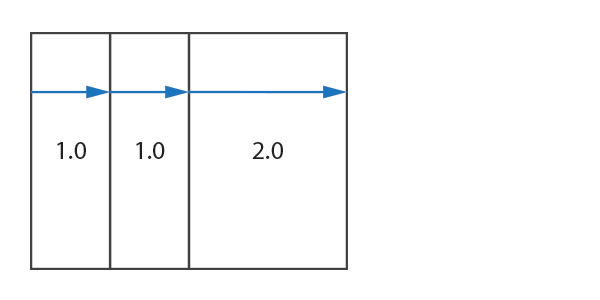The element with the `2.0` stretch value stretches twice as much compared to its siblings.

### vstretchOverrides the vertical stretchiness of an an enclosed element (`subject`).

#### Expression

``````vstretch(stretch, subject)
``````

#### Notation

 `stretch` A scalar value `subject` Instance of `Element`

#### Semantics

1. The `subject` will assume the given `stretch` value.
2. The stretch value has no effect to elements with fixed vertical limits.
3. Returns instance of `Proxy`.

For example, the image below shows how three elements are laid out in an `htile`, with stretch values of `0.5`, `1.0` and `1.5`, respectively: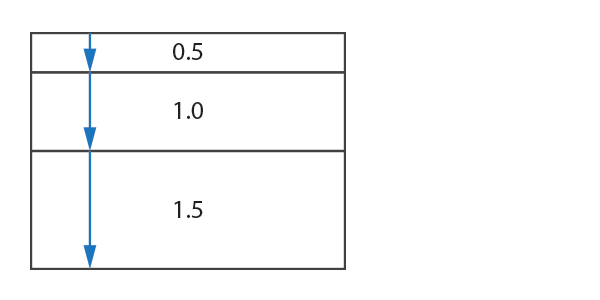The element with the `0.5` stretch value stretches half less, while the element with the `1.5` stretches half more than the default.

## Scale Element

The Elements Library is resolution independent and elements can be scaled up or down to suit. The default scale is 1.0 (no scale).

### scale

The `scale` element changes the scale of its enclosed element (`subject`).

#### Expression

``````scale(scale_, subject)
``````

#### Notation

 `scale_` `float` `subject` Instance of `Element`

#### Semantics

1. The `subject` will scaled given the `scale_` value. A value > 1.0 scales the element up (zoom in), while a value < 1.0 scales down (zoom out).
2. Returns instance of `Proxy`.

## Align Elements

An element can be aligned arbitrarily from 0.0 to 1.0 in either the x or y dimensions, or both. There is a comprehensive list of align elements in The Elements Library that can be used for various purposes. This section catalogues all the available align elements.

### halign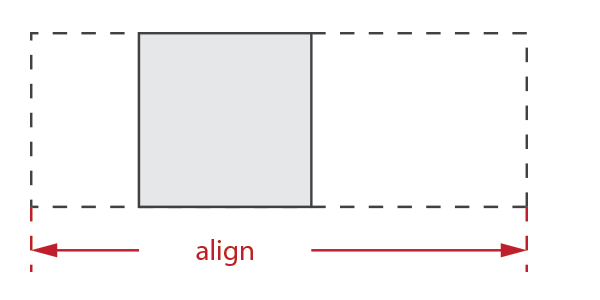Aligns the an enclosed element (`subject`) in the x-axis.

#### Expression

``````halign(align, subject)
``````

#### Notation

 `align` `float` `subject` Instance of `Element`

#### Semantics

1. Given a total allocated space `X`, `subject` will be positioned horizontally to `X * align`.
2. The `subject` will assume its minimum horizontal limit.
3. Returns instance of `Proxy`.

#### Examples

1. `halign(0.0) // align subject to the left`
2. `halign(1.0) // align subject to the right`
3. `halign(0.5) // align subject to the center`

### align_left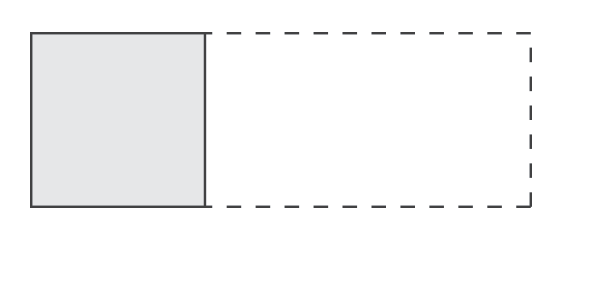Left-aligns the an enclosed element (`subject`).

#### Expression

``````align_left(subject)
``````

#### Notation

 `subject` Instance of `Element`

#### Semantics

1. Equivalent to `halign(0.0, subject)`
2. Returns instance of `Proxy`.

### align_center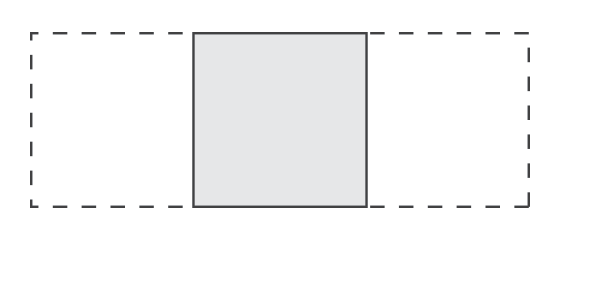Center-aligns the an enclosed element (`subject`).

#### Expression

``````align_center(subject)
``````

#### Notation

 `subject` Instance of `Element`

#### Semantics

1. Equivalent to `halign(0.5, subject)`
2. Returns instance of `Proxy`.

### align_rightRight-aligns the an enclosed element (`subject`).

#### Expression

``````align_right(subject)
``````

#### Notation

 `subject` Instance of `Element`

#### Semantics

1. Equivalent to `halign(1.0, subject)`
2. Returns instance of `Proxy`.

### valign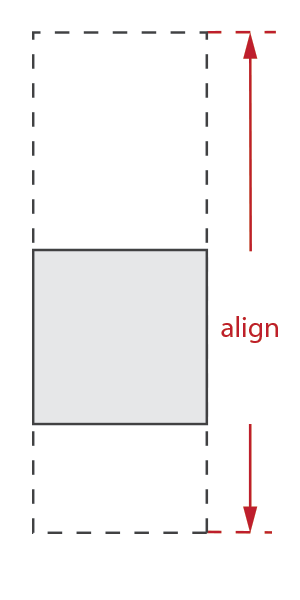Aligns the an enclosed element (`subject`) in the y-axis.

#### Expression

``````valign(align, subject)
``````

#### Notation

 `align` `float` `subject` Instance of `Element`

#### Semantics

1. Given a total allocated space `Y`, `subject` will be positioned vertically to `Y * align`.
2. The `subject` will assume its minimum vertical limit.
3. Returns instance of `Proxy`.

#### Examples

1. `valign(0.0) // align subject to the top`
2. `valign(1.0) // align subject to the right`
3. `valign(0.5) // align subject to the middle`

### align_topAligns the an enclosed element (`subject`) to the top.

#### Expression

``````align_top(subject)
``````

#### Notation

 `subject` Instance of `Element`

#### Semantics

1. Equivalent to `valign(0.0, subject)`
2. Returns instance of `Proxy`.

### align_middle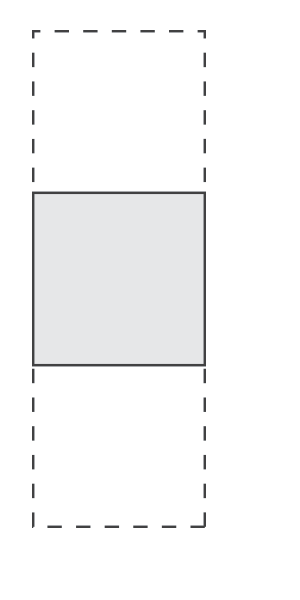Aligns the an enclosed element (`subject`) to the middle.

#### Expression

``````align_middle(subject)
``````

#### Notation

 `subject` Instance of `Element`

#### Semantics

1. Equivalent to `valign(0.5, subject)`
2. Returns instance of `Proxy`.

### align_bottomAligns the an enclosed element (`subject`) to the bottom.

#### Expression

``````align_bottom(subject)
``````

#### Notation

 `subject` Instance of `Element`

#### Semantics

1. Equivalent to `valign(1.0, subject)`
2. Returns instance of `Proxy`.

### align_left_topAligns the an enclosed element (`subject`) to the left-top.

#### Expression

``````align_left_top(subject)
``````

#### Notation

 `subject` Instance of `Element`

#### Semantics

1. Equivalent to `align_left(align_top(subject))`
2. Returns instance of `Proxy`.

### align_center_top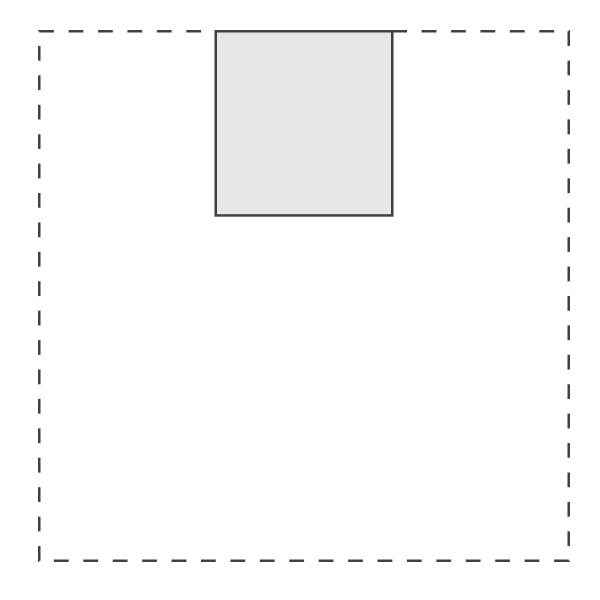Aligns the an enclosed element (`subject`) to the center-top.

#### Expression

``````align_center_top(subject)
``````

#### Notation

 `subject` Instance of `Element`

#### Semantics

1. Equivalent to `align_center(align_top(subject))`
2. Returns instance of `Proxy`.

### align_right_top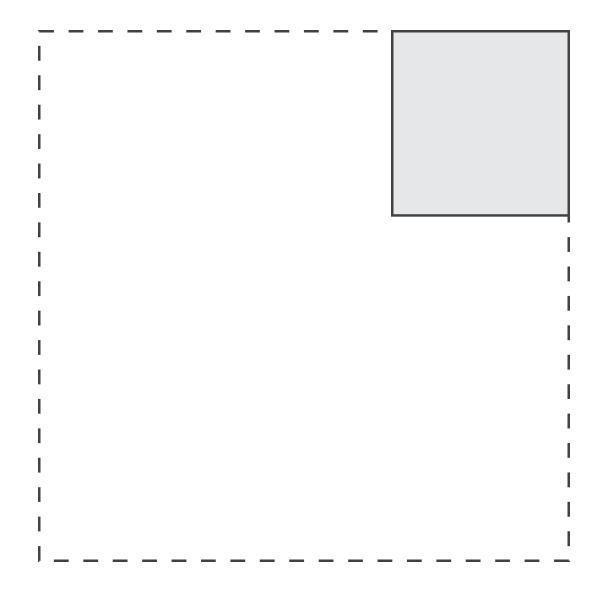Aligns the an enclosed element (`subject`) to the right-top.

#### Expression

``````align_right_top(subject)
``````

#### Notation

 `subject` Instance of `Element`

#### Semantics

1. Equivalent to `align_right(align_top(subject))`
2. Returns instance of `Proxy`.

### align_left_middle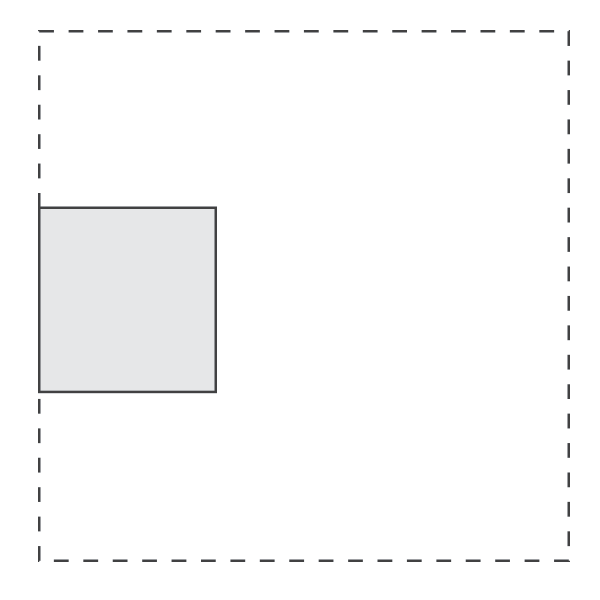Aligns the an enclosed element (`subject`) to the left-middle.

#### Expression

``````align_left_middle(subject)
``````

#### Notation

 `subject` Instance of `Element`

#### Semantics

1. Equivalent to `align_left(align_middle(subject))`
2. Returns instance of `Proxy`.

### align_center_middle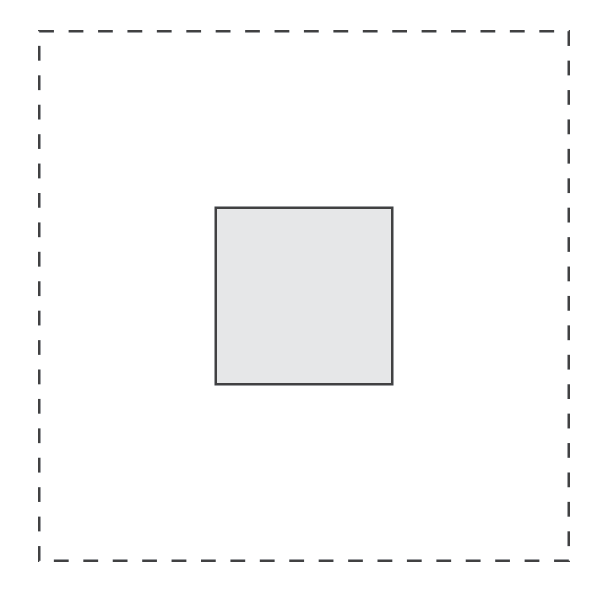Aligns the an enclosed element (`subject`) to the center-middle.

#### Expression

``````align_center_middle(subject)
``````

#### Notation

 `subject` Instance of `Element`

#### Semantics

1. Equivalent to `align_center(align_middle(subject))`
2. Returns instance of `Proxy`.

### align_right_middle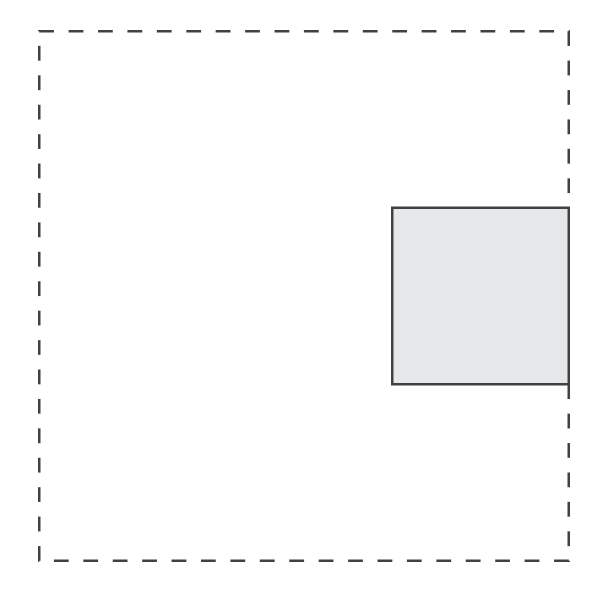Aligns the an enclosed element (`subject`) to the right-middle.

#### Expression

``````align_right_middle(subject)
``````

#### Notation

 `subject` Instance of `Element`

#### Semantics

1. Equivalent to `align_right(align_middle(subject))`
2. Returns instance of `Proxy`.

### align_left_bottomAligns the an enclosed element (`subject`) to the left-bottom.

#### Expression

``````align_left_bottom(subject)
``````

#### Notation

 subject Instance of `Element`

#### Semantics

1. Equivalent to `align_left(align_bottom(subject))`
2. Returns instance of `Proxy`.

### align_center_bottomAligns the an enclosed element (`subject`) to the center-bottom.

#### Expression

``````align_center_bottom(subject)
``````

#### Notation

 `subject` Instance of `Element`

#### Semantics

1. Equivalent to `align_center(align_bottom(subject))`
2. Returns instance of `Proxy`.

### align_right_bottom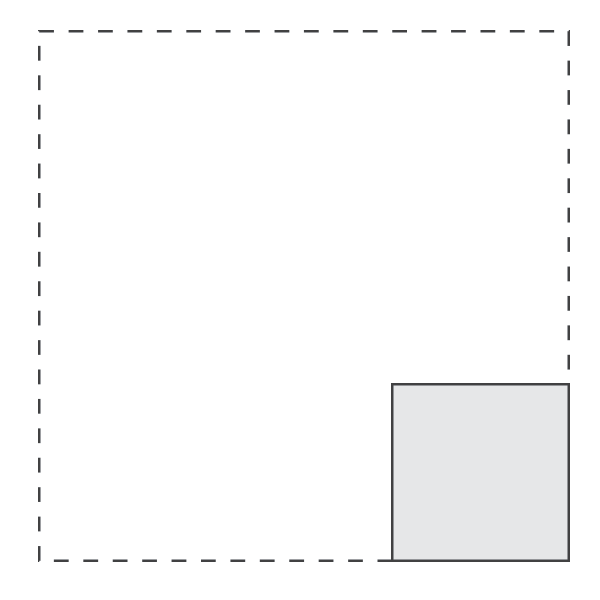Aligns the an enclosed element (`subject`) to the right-bottom.

#### Expression

``````align_right_bottom(subject)
``````

#### Notation

 `subject` Instance of `Element`

#### Semantics

1. Equivalent to `align_right(align_bottom(subject))`
2. Returns instance of `Proxy`.

## Margin Elements

Margins add some space around elements. There is a comprehensive list of margin elements in the Elements Library that can be used for various purposes. This section catalogues all the available margin elements.

### margin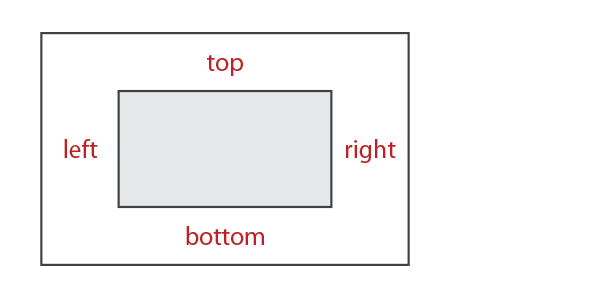Adds a margin all around an enclosed element (`subject`).

#### Expression

``````margin({ left, top, right, bottom }, subject)
``````

#### Notation

 `left`, `top`, `right`, `bottom` `float` `subject` Instance of `Element`

#### Semantics

1. Space is added to the left, top, right, and bottom of the subject with the given parameters.
2. The element’s limits is overridden to account for the additional space.
3. The `margin` does not violate the natural limits of the subject. `margin` will respect the subject’s min-max constraints and resizability.
4. Returns instance of `Proxy`.

### left_marginAdds a margin to the left of an enclosed element (`subject`).

#### Expression

``````left_margin(left, subject)
``````

#### Notation

 `left` `float` `subject` Instance of `Element`

#### Semantics

1. Space is added to the left of the subject with the given parameter.
2. The element’s limits is overridden to account for the additional space.
3. The `left_margin` does not violate the natural limits of the subject. `left_margin` will respect the subject’s min-max constraints and resizability.
4. Returns instance of `Proxy`.

### right_marginAdds a margin to the right of an enclosed element (`subject`).

#### Expression

``````right_margin(right, subject)
``````

#### Notation

 `right` `float` `subject` Instance of `Element`

#### Semantics

1. Space is added to the right of the subject with the given parameter.
2. The element’s limits is overridden to account for the additional space.
3. The `right_margin` does not violate the natural limits of the subject. `right_margin` will respect the subject’s min-max constraints and resizability.
4. Returns instance of `Proxy`.

### top_marginAdds a margin to the top of an enclosed element (`subject`).

#### Expression

``````top_margin(top, subject)
``````

#### Notation

 `top` `float` `subject` Instance of `Element`

#### Semantics

1. Space is added to the top of the subject with the given parameter.
2. The element’s limits is overridden to account for the additional space.
3. The `top_margin` does not violate the natural limits of the subject. `top_margin` will respect the subject’s min-max constraints and resizability.
4. Returns instance of `Proxy`.

### bottom_marginAdds a margin to the bottom of an enclosed element (`subject`).

#### Expression

``````bottom_margin(bottom, subject)
``````

#### Notation

 `bottom` `float` `subject` Instance of `Element`

#### Semantics

1. Space is added to the to the bottom of the subject with the given parameter.
2. The element’s limits is overridden to account for the additional space.
3. The `bottom_margin` does not violate the natural limits of the subject. `bottom_margin` will respect the subject’s min-max constraints and resizability.
4. Returns instance of `Proxy`.

### hmargin

(same as `left_right_margin`)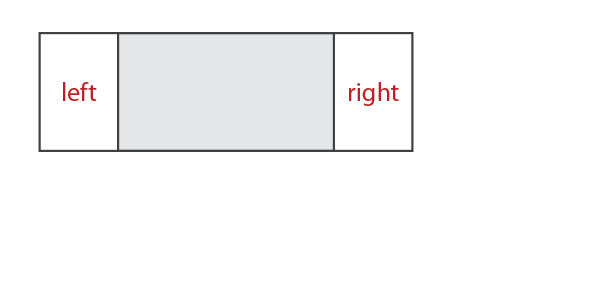Adds a margin to the left and right sides of an enclosed element (`subject`).

#### Expressions

``````// variant 1
hmargin({ left, right }, subject)

// variant 2
hmargin(left, right, subject)

// variant 3
left_right_margin({ left, right }, subject)

// variant 4
left_right_margin(left, right, subject)
``````

#### Notation

 `left`, `right` `float` `subject` Instance of `Element`

#### Semantics

1. Space is added to the to the left and right sides of the subject with the given parameters.
2. The element’s limits is overridden to account for the additional space.
3. The `hmargin` (and variants) does not violate the natural limits of the subject. `hmargin` (and variants) will respect the subject’s min-max constraints and resizability.
4. Returns instance of `Proxy`.

### vmargin

(same as `top_bottom_margin`)Adds a margin to the top and bottom sides of an enclosed element (`subject`).

#### Expressions

``````// variant 1
vmargin({ top, bottom }, subject)

// variant 2
vmargin(top, bottom, subject)

// variant 3
top_bottom_margin({ top, bottom }, subject)

// variant 4
top_bottom_margin(top, bottom, subject)
``````

#### Notation

 `top`, `bottom` `float` `subject` Instance of `Element`

#### Semantics

1. Space is added to the to the top and bottom sides of the subject with the given parameters.
2. The element’s limits is overridden to account for the additional space.
3. The `vmargin` (and variants) does not violate the natural limits of the subject. `vmargin` (and variants) will respect the subject’s min-max constraints and resizability.
4. Returns instance of `Proxy`.

### left_top_marginAdds a margin to the left and top sides of an enclosed element (`subject`).

#### Expressions

``````// variant 1
left_top_margin({ left, top }, subject)

// variant 2
left_top_margin(left, top, subject)
``````

#### Notation

 `left`, `top` `float` `subject` Instance of `Element`

#### Semantics

1. Space is added to the to the left and top sides of the subject with the given parameters.
2. The element’s limits is overridden to account for the additional space.
3. The `left_top_margin` (and variant) does not violate the natural limits of the subject. `left_top_margin` (and variant) will respect the subject’s min-max constraints and resizability.
4. Returns instance of `Proxy`.

### left_bottom_margin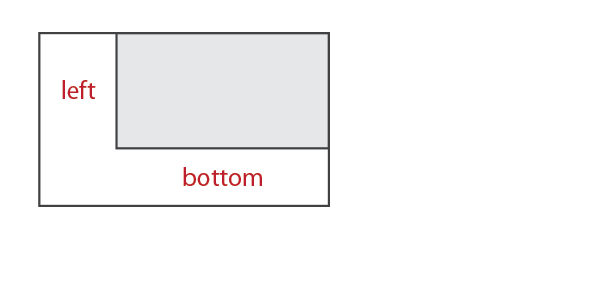Adds a margin to the left and bottom sides of an enclosed element (`subject`).

#### Expressions

``````// variant 1
left_bottom_margin({ left, bottom }, subject)

// variant 2
left_bottom_margin(left, bottom, subject)
``````

#### Notation

 `left`, `bottom` `float` `subject` Instance of `Element`

#### Semantics

1. Space is added to the to the left and bottom sides of the subject with the given parameters.
2. The element’s limits is overridden to account for the additional space.
3. The `left_bottom_margin` (and variant) does not violate the natural limits of the subject. `left_bottom_margin` (and variant) will respect the subject’s min-max constraints and resizability.
4. Returns instance of `Proxy`.

### right_top_margin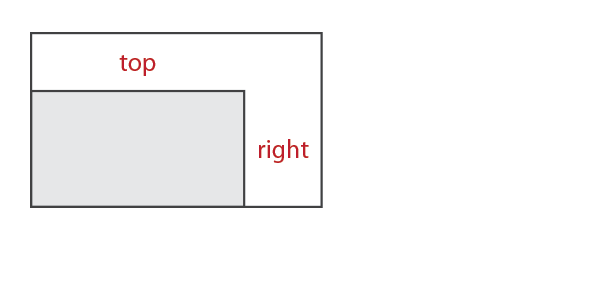Adds a margin to the right and top sides of an enclosed element (`subject`).

#### Expressions

``````// variant 1
right_top_margin({ right, top }, subject)

// variant 2
right_top_margin(right, top, subject)
``````

#### Notation

 `right`, `top` `float` `subject` Instance of `Element`

#### Semantics

1. Space is added to the to the right and top sides of the subject with the given parameters.
2. The element’s limits is overridden to account for the additional space.
3. The `right_top_margin` (and variant) does not violate the natural limits of the subject. `right_top_margin` (and variant) will respect the subject’s min-max constraints and resizability.
4. Returns instance of `Proxy`.

### right_bottom_marginAdds a margin to the right and bottom sides of an enclosed element (`subject`).

#### Expressions

``````// variant 1
right_bottom_margin({ right, bottom }, subject)

// variant 2
right_bottom_margin(right, bottom, subject)
``````

#### Notation

 `right`, `bottom` `float` `subject` Instance of `Element`

#### Semantics

1. Space is added to the to the right and bottom sides of the subject with the given parameters.
2. The element’s limits is overridden to account for the additional space.
3. The `right_bottom_margin` (and variant) does not violate the natural limits of the subject. `right_bottom_margin` (and variant) will respect the subject’s min-max constraints and resizability.
4. Returns instance of `Proxy`.

## Floating ElementA floating element allows arbitrary placement of an enclosed element (`subject`) in the main view.

#### Expression

``````floating({ left, top, right, bottom }, subject)
``````

#### Notation

 `left`, `top`, `right`, `bottom` `float` `subject` Instance of `Element`

#### Semantics

1. The element will be placed exactly to the specified position in the main view, constrained to the subject’s natural limits.
2. The floating element does not violate the natural limits of the subject.
3. Returns instance of `Proxy`.

## Tiles and Grids

Tiles are the most useful layout elements, followed by by Grids. Tiles are used everywhere for composing hierarchical elements in rows and columns, typical to all GUIs. Grids are similar to tiles. Both tiles and grids allow elements to fluidly adjust depending on available space. Tiles compute the layout of its elements using the children elements’ size `limits` while grids lay out its elements using an externally supplied fractional coordinates that specify positions of the elements in the allocated space.

Tiles are best used for composing UI elements while grids are best for composing tables.

### Horizontal Grids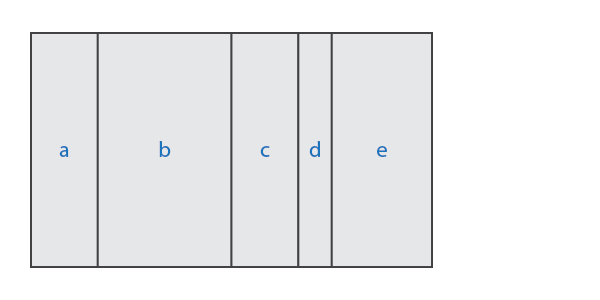Horizontal Grids are composites that lay out one or more child elements in a row following externally supplied horizontal fractional positions. Horizontal Grids have computed horizontal and vertical sizes following the natural `limits` of its children.

#### Semantics

1. The elements are laid out in a single row, left to right, immediately next to each other with no intervening space.
2. The elements are positioned horizontally using the supplied fractional positions. The fractional positions values range from 0.0 to 1.0, which specify the child element’s horizontal position from left (0.0) to right (1.0).
3. The grid’s minimum vertical limit is computed as the maximum of the children elements’ minimum vertical limits.
4. The grid’s maximum vertical limit is computed as the minimum of the children elements’ maximum vertical limits.
5. The final computed minimum limit is clamped to ensure it is not greater than the computed maximum limit. Likewise the computed maximum limit is clamped to ensure it is not less than the computed minimum limit.
6. The supplied (horizontal) positions and computed (vertical) coordinates may violate the limits of its children elements.
1. If the allocated size of a child element is lower than the element’s minimum limits in either dimension, the element will be cropped.
2. If a child element’s maximum limits in either dimension is exceeded, the element will be aligned to the top-left.

### hgrid

Build a horizontal grid with a fixed number of elements.

#### Expression

``````hgrid(positions, e1,...eN)
``````

#### Notation

 `N` The number of items `e1,...eN` One or more child elements, instances of `Element` (more below) `positions` External container of fractional positions (more below)

The external container, `positions`, can either be a plain array of type `float[N]` or `std::array<float, N>`. Elements `e1,...eN` are held in a `std::array<element_ptr, N>` managed by the horizontal grid element.

#### Example

``````static float positions[] = { 0.25, 0.5, 0.75, 1.0 };
//...
hgrid(positions, item1, item2, item3, item4)
``````If the number of elements is not fixed, you can use an `hgrid_composite` (see below).

#### Requirements

1. The number of supplied positions and elements should match, otherwise, compiler error (no matching function for call to `hgrid`).
2. The positions assume the first element is at `x=0` (it is at the left-most position in the row). The fractional position of the second element is at index `0`, the third at index `1`, and so on.
3. The externally supplied positions should be sorted with increasing values such that positions[n] <= positions[n+1]. The behavior is undefined if this is violated.

#### Semantics

1. In addition to the semantics of Horizontal Grids, returns instance of `Composite`.

### hgrid_composite

Create a horizontal grid with an indeterminate (dynamic) number of elements.

#### Expression

``````hgrid_composite c{ positions };
``````

#### Notation

 `positions` External container of fractional positions, `std::vector` `c` Instance of type `hgrid_composite`

The `hgrid_composite` is basically a `std::vector<element_ptr>` that the client uses to manage the composite’s elements. The lifetime of the container, `c`, is the client’s responsibility. You use `hgrid_composite` just as you would a `std::vector`, such as `push_back` a child element. Just keep in mind that we are dealing with `element_ptr` items.

#### Example

``````c.push_back(share(child));
```````share` turns an element object into an `element_ptr` held by the `std::vector<element_ptr>` in `hgrid_composite`.

`hgrid_composite` is itself also an `element` and while it has `std::vector`’s interface, it can also be `share`d like any element, which allows you to build complex hierarchical structures.

#### Requirements

1. The number of items in the external coordinates vector `positions` must match with the number of elements at any given time.
2. The positions assume the first element is at `x=0` (it is at the left-most position in the row). The fractional position of the second element is at index `0`, the third at index `1`, and so on.
3. The externally supplied positions should be sorted with increasing values such that positions[n] <= positions[n+1]. The behavior is undefined if this is violated.

### Horizontal TilesHorizontal Tiles are similar to Horizontal Grids, but allow elements to fluidly adjust horizontally depending on available space. Horizontal Tiles are best used for composing UI elements while Horizontal Grids are best for composing tables.

#### Semantics

1. The elements are laid out in a single row, left to right, immediately next to each other with no intervening space.
2. The elements are positioned horizontally using the children’s natural limits.
3. Horizontal space is allocated using this algorithm:
1. Space is allocated for each child element following the child’s natural minimum horizontal limit.
2. If the allocated space exceeds the sum of all children elements’ minimum horizontal limits, the extra space is given to each horizontally resizable element (`limits.min.x < limits.max.x`).
3. The element’s “stretchiness” determines how much extra space is given to it according to the element’s `stretch()` member function. A stretch value of `1.0` is default. A stretchiness value of 2.0 means that the element is able to stretch twice as much compared to its siblings. Horizontally fixed-sized elements will not be stretched (element d in the diagram). (Also see Stretch Elements).
4. The tile’s minimum vertical limit is computed as the maximum of the children elements’ minimum vertical limits.
5. The grid’s maximum vertical limit is computed as the minimum of the children elements’ maximum vertical limits.
6. The final computed minimum limit is clamped to ensure it is not greater than the computed maximum limit. Likewise the computed maximum limit is clamped to ensure it is not less than the computed minimum limit.
7. The supplied (horizontal) and computed (vertical) coordinates may violate the limits of its children elements.
1. If the allocated size of a child element is lower than the element’s minimum limits in either dimension, the element will be cropped.
2. If a child element’s maximum limits in either dimension is exceeded, the element will be aligned to the top-left.

### htile

Build a horizontal tile with a fixed number of elements.

#### Expression

``````htile(e1,...eN)
``````

#### Notation

 `N` The number of items `e1,...eN` One or more child elements, instances of `Element` (more below)

Elements `e1,...eN` are held in a `std::array<element_ptr, N>` managed by the horizontal tile element.

#### Example:

``````htile(item1, item2, item3, item4)
``````If the number of elements is not fixed, you can use an `htile_composite` (see below).

#### Semantics

1. In addition to the semantics of Horizontal Tiles, returns instance of `Composite`.

### htile_composite

Create a horizontal tile with an indeterminate (dynamic) number of elements.

#### Expression

``````htile_composite c;
``````

#### Notation

 `c` Instance of type `htile_composite`

The `htile_composite` is basically a `std::vector<element_ptr>` that the client uses to manage the composite’s elements. The lifetime of the container, `c`, is the client’s responsibility. You use `htile_composite` just as you would a `std::vector`, such as `push_back` a child element. Just keep in mind that we are dealing with `element_ptr` items.

#### Example

``````c.push_back(share(child));
```````share` turns an element object into an `element_ptr` held by the `std::vector<element_ptr>` in `htile_composite`.

`htile_composite` is itself also an `element` and while it has `std::vector`’s interface, it can also be `share`d like any element, which allows you to build complex hierarchical structures.

### Vertical Grids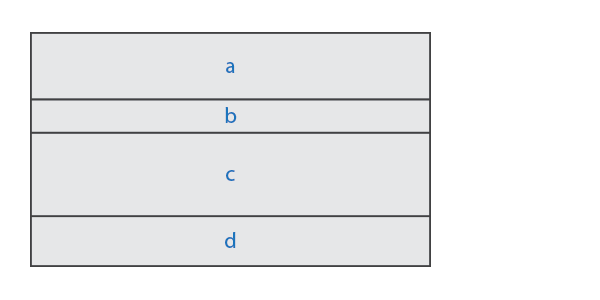Vertical Grids are composites that lay out one or more child elements in a column following externally supplied vertical fractional positions. Vertical Grids have computed horizontal and vertical sizes following the natural `limits` of its children.

#### Semantics

1. The elements are laid out in a single column, top to bottom, immediately next to each other with no intervening space.
2. The elements are positioned vertically using the supplied fractional positions. The fractional positions values range from 0.0 to 1.0, which specify the child element’s vertical position from top (0.0) to bottom (1.0).
3. The grid’s minimum horizontal limit is computed as the maximum of the children elements’ minimum horizontal limits.
4. The grid’s maximum horizontal limit is computed as the minumum of the children elements’ maximum horizontal limits.
5. The final computed minimum limit is clamped to ensure it is not greater than the computed maximum limit. Likewise the computed maximum limit is clamped to ensure it is not less than the computed minimum limit.
6. The supplied (vertical) positions and computed (horizontal) coordinates may violate the limits of its children elements.
1. If the allocated size of a child element is lower than the element’s minimum limits in either dimension, the element will be cropped.
2. If a child element’s maximum limits in either dimension is exceeded, the element will be aligned to the top-left.

### vgrid

Build a vertical grid with a fixed number of elements.

#### Expression

``````vgrid(positions, e1,...eN)
``````

#### Notation

 `N` The number of items `e1,...eN` One or more child elements, instances of `Element` (more below) `positions` External container of fractional positions (more below)

The External container, `positions` is an external can either be a plain array of type `float[N]` or `std::array<float, N>`. Elements `e1,...eN` are held in a `std::array<element_ptr, N>` managed by the vertical grid element.

#### Example

``````static float positions[] = { 0.25, 0.5, 0.75, 1.0 };
//...
vgrid(positions, item1, item2, item3, item4)
``````If the number of elements is not fixed, you can use an `vgrid_composite` (see below).

#### Requirements

1. The number of supplied coordinates and elements should match, otherwise, compiler error (no matching function for call to `vgrid`).
2. The positions assume the first element is at `x=0` (it is at the top-most position in the column). The fractional position of the second element is at index `0`, the third at index `1`, and so on.
3. The externally supplied positions should be sorted with increasing values such that positions[n] <= positions[n+1]. The behavior is undefined if this is violated.

#### Semantics

1. In addition to the semantics of Vertical Grids, returns instance of `Composite`.

### vgrid_composite

Create a vertical grid with an indeterminate (dynamic) number of elements.

#### Expression

``````vgrid_composite c{ positions };
``````

#### Notation

 `positions` External container of fractional positions, `std::vector` `c` Instance of type `vgrid_composite`

The `vgrid_composite` is basically a `std::vector<element_ptr>` that the client uses to manage the composite’s elements. The lifetime of the container, `c`, is the client’s responsibility. You use `vgrid_composite` just as you would a `std::vector`, such as `push_back` a child element. Just keep in mind that we are dealing with `element_ptr` items. Example:

``````c.push_back(share(child));
```````share` turns an element object into an `element_ptr` held by the `std::vector<element_ptr>` in `vgrid_composite`.

`vgrid_composite` is itself also an `element` and while it has `std::vector`’s interface, it can also be `share`d like any element, which allows you to build complex hierarchical structures.

#### Requirements

1. The number of items in the external coordinates vector `positions` must match with the number of elements at any given time.
2. The positions assume the first element is at `x=0` (it is at the top-most position in the column). The fractional position of the second element is at index `0`, the third at index `1`, and so on.
3. The externally supplied positions should be sorted with increasing values such that positions[n] <= positions[n+1]. The behavior is undefined if this is violated.

### Vertical TilesVertical Tiles are similar to Vertical Grids, but allow elements to fluidly adjust vertically depending on available space. Vertical Tiles are best used for composing UI elements while Vertical Grids are best for composing tables.

#### Semantics

1. The elements are laid out in a single column, left to right, immediately next to each other with no intervening space.
2. The elements are positioned vertically using the children’s natural limits.
3. Vertical space is allocated using this algorithm:
1. Space is allocated for each child element following the child’s natural minimum vertical limit.
2. If the allocated space exceeds the sum of all children elements’ minimum vertical limits, the extra space is given to each vertically resizable element (`limits.min.y < limits.max.y`).
3. The element’s “stretchiness” determines how much extra space is given to it according to the element’s `stretch()` member function. A stretch value of `1.0` is default. A stretchiness value of 2.0 means that the element is able to stretch twice as much compared to its siblings. Vertically fixed-sized elements will not be stretched (element b in the diagram). (Also see Stretch Elements).
4. The tile’s minimum horizontal limit is computed as the maximum of the children elements’ minimum horizontal limits.
5. The grid’s maximum horizontal limit is computed as the minumum of the children elements’ maximum horizontal limits.
6. The final computed minimum limit is clamped to ensure it is not greater than the computed maximum limit. Likewise the computed maximum limit is clamped to ensure it is not less than the computed minimum limit.
7. The supplied (vertical) and computed (horizontal) coordinates may violate the limits of its children elements.
1. If the allocated size of a child element is lower than the element’s minimum limits in either dimension, the element will be cropped.
2. If a child element’s maximum limits in either dimension is exceeded, the element will be aligned to the top-left.

### vtile

Build a vertical tile with a fixed number of elements.

#### Expression

``````vtile(e1,...eN)
``````

#### Notation

 `N` The number of items `e1,...eN` One or more child elements, instances of `Element` (more below)

Elements `e1,...eN` are held in a `std::array<element_ptr, N>` managed by the vertical tile element.

#### Example

``````vtile(item1, item2, item3, item4)
``````If the number of elements is not fixed, you can use an `vtile_composite` (see below).

#### Semantics

1. In addition to the semantics of Vertical Tiles, returns instance of `Composite`.

### vtile_composite

Create a vertical tile with an indeterminate (dynamic) number of elements:

#### Expression

``````vtile_composite c;
``````

#### Notation

 `c` Instance of type `vtile_composite`

The `vtile_composite` is basically a `std::vector<element_ptr>` that the client uses to manage the composite’s elements. The lifetime of the container, `c`, is the client’s responsibility. You use `vtile_composite` just as you would a `std::vector`, such as `push_back` a child element. Just keep in mind that we are dealing with `element_ptr` items.

#### Example

``````c.push_back(share(child));
```````share` turns an element object into an `element_ptr` held by the `std::vector<element_ptr>` in `vtile_composite`.

`vtile_composite` is itself also an `element` and while it has `std::vector`’s interface, it can also be `share`d like any element, which allows you to build complex hierarchical structures.

## LayersThe Elements Library is 2D, but the z-axis pertains to top-to-bottom layering. Layers allow groups of elements to be placed in the z-axis where higher-level elements obscure or hide lower-level elements.

#### Semantics

1. The elements are laid out in the z-axis, top to bottom.
2. Rendering is done bottom-up; the bottom-most elements are drawn first.
3. UI control (such as mouse clicks) proceeds from top to bottom. Higher-level elements are given control priority. If a higher-level element does not process the event, lower-level elements are given a chance.
4. The layer’s minimum limit is computed as the maximum of the children elements’ minimum limits.
5. The layer’s maximum limit is computed as the minimum of the children elements’ maximum limits.
6. The final computed minimum limit is clamped to ensure it is not greater than the computed maximum limit. Likewise the computed maximum limit is clamped to ensure it is not less than the computed minimum limit.
7. The computed (vertical) and (horizontal) coordinates may violate the limits of its children elements.
1. If the allocated size of a child element is lower than the element’s minimum limits in either dimension, the element will be cropped.
2. If a child element’s maximum limits in either dimension is exceeded, the element will be aligned to the top-left.

### layer

Create a layer composite with a fixed number of elements.

#### Expression

``````layer(e1,...eN)
``````

#### Notation

 `N` The number of items `e1,...eN` One or more child elements, instances of `Element` (more below)

Elements `e1,...eN` are held in a `std::array<element_ptr, N>` managed by the layer element.

#### Example

``````layer(item1, item2, item3, item4)
``````If the number of elements is not fixed, you can use an `layer_composite` (see below).

#### Semantics

1. In addition to the semantics of Layers, returns instance of `Composite`.

### layer_composite

Create a layer with an indeterminate (dynamic) number of elements:

``````layer_composite c;
``````

#### Notation

 `c` Instance of type `layer_composite`

The `layer_composite` is basically a `std::vector<element_ptr>` that the client uses to manage the composite’s elements. The lifetime of the container, `c`, is the client’s responsibility. You use `layer_composite` just as you would a `std::vector`, such as `push_back` a child element. Just keep in mind that we are dealing with `element_ptr` items.

#### Example

``````c.push_back(share(child));
```````share` turns an element object into an `element_ptr` held by the `std::vector<element_ptr>` in `layer_composite`.

`layer_composite` is itself also an `element` and while it has `std::vector`’s interface, it can also be `share`d like any element, which allows you to build complex hierarchical structures.

### Decks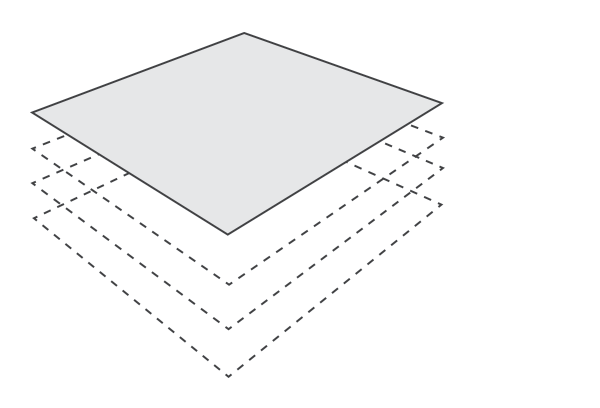The Deck is very similar to layers. Elements are placed in the z-axis. But unlike layers, only selected element is active (top-most by default).

#### Semantics

1. Everything listed in the layer’s Semantics, except 2 and 3.
2. Only the active selected element element is drawn.
3. Only the active selected element is given the chance to process UI control.

### deck

Create a deck composite with a fixed number of elements.

#### Expression

``````deck(e1, e2, e3... eN)
``````

#### Notation

 `N` The number of items `e1,...eN` One or more child elements, instances of `Element` (more below)

Elements `e1,...eN` are held in a `std::array<element_ptr, N>` managed by the deck element.

#### Example

``````deck(item1, item2, item3, item4)
``````If the number of elements is not fixed, you can use an `deck_composite` (see below).

#### Requirements

1. In addition to the semantics of Deck, returns instance of `Composite`.

### deck_composite

Create a deck with an indeterminate (dynamic) number of elements:

#### Expression

``````deck_composite c;
``````

#### Notation

 `c` Instance of type `deck_composite`

The `deck_composite` is basically a `std::vector<element_ptr>` that the client uses to manage the composite’s elements. The lifetime of the container, `c`, is the client’s responsibility. You use `deck_composite` just as you would a `std::vector`, such as `push_back` a child element. Just keep in mind that we are dealing with `element_ptr` items.

#### Example

``````c.push_back(share(child));
```````share` turns an element object into an `element_ptr` held by the `std::vector<element_ptr>` in `deck_composite`.

`deck_composite` is itself also an `element` and while it has `std::vector`’s interface, it can also be `share`d like any element, which allows you to build complex hierarchical structures.

## Flow Element

The flow element, is a composite that lays out its children much like the way text is laid out: lay out each element from left to right, fitting as much elements as possible following each child’s maximum horizontal limit. Once a row is full, move to the next row and do the same until the end of the row is filled. Repeat the procedure until all the elements are laid out. The height of each row is determined by the maximum vertical limit of all the elements to be laid out in that row. The following graphic depicts a simplified layout scenario for child elements `a` to `r`.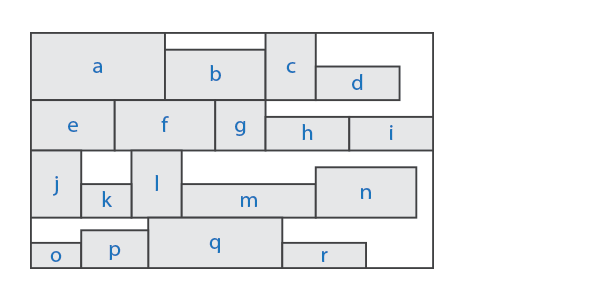The child elements arranged in a `flow` composite are automatically re-flowed (re-lay-out) when the view size changes.

To have elements laid out using `flow`, you need to make a `flow_composite`.

### flow_composite

Create a `flow_composite` with an indeterminate (dynamic) number of elements.

#### Expression

``````flow_composite c;
``````

#### Notation

 `c` Instance of type `flow_composite`

The `flow_composite` is basically a `std::vector<element_ptr>` that the client uses to manage the composite’s elements. The lifetime of the container, `c`, is the client’s responsibility. You use `flow_composite` just as you would a `std::vector`, such as `push_back` a child element, `child`. Just keep in mind that we are dealing with `element_ptr` items.

#### Example

``````c.push_back(share(child));
```````share` turns an element object into an `element_ptr` held by the `std::vector<element_ptr>` in `flow_composite`.

`flow_composite` is itself also an `element` and while it has `std::vector`’s interface, it can also be `share`d like any element, which allows you to build complex hierarchical structures.

### flow

Once we have a `flow_composite`, we can place its contents in a `flow` element.

#### Expression

``````flow(c)
``````

#### Notation

 `c` Instance of `flow_composite`

#### Semantics

1. Returns instance of `Composite`.